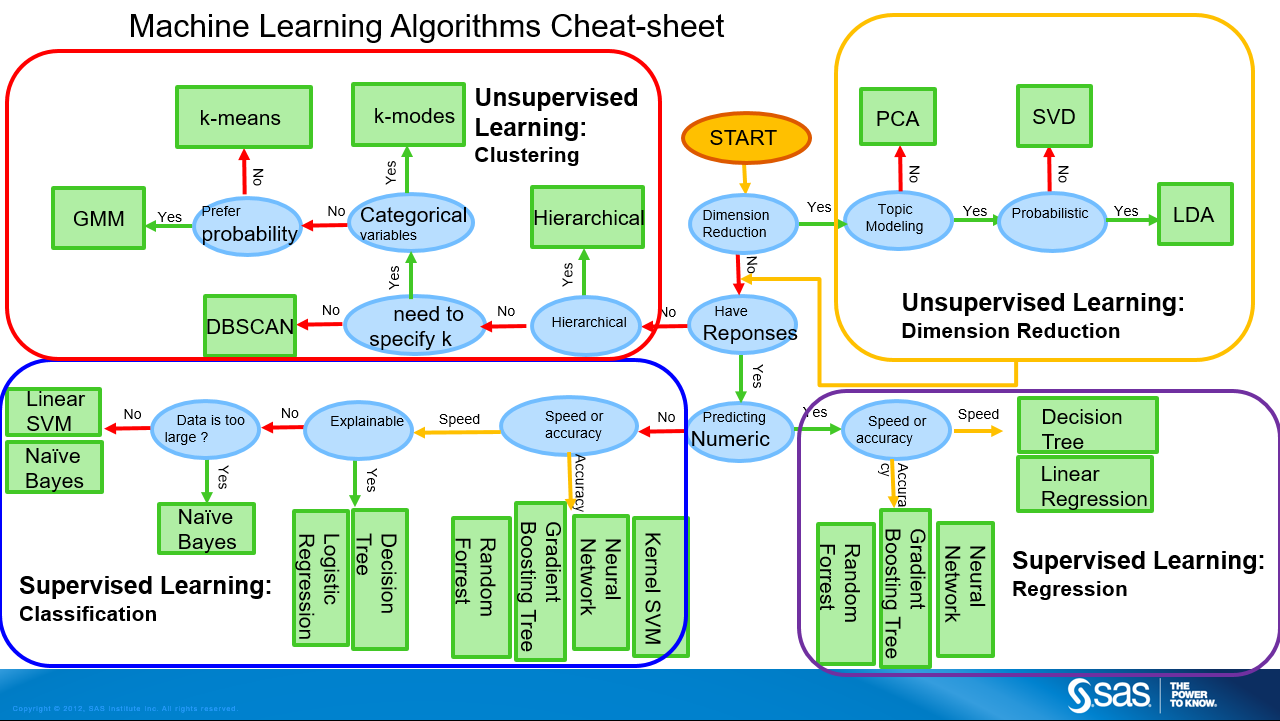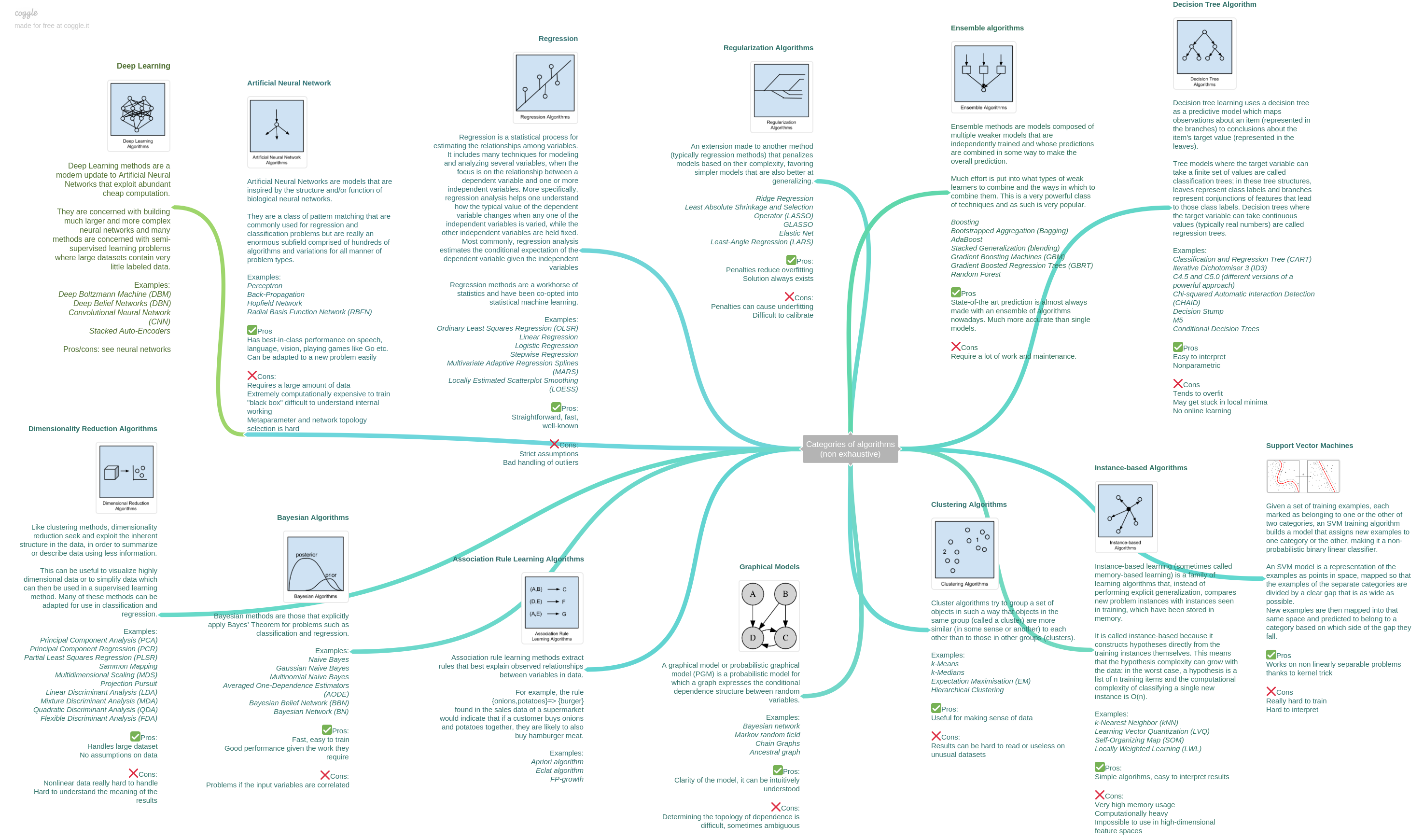# 算法列表(图片来自 blogs.sas.com)

## 算法列表

• Regularization Algorithms: An extension made to another method (typically regression methods) that penalizes models based on their complexity, favoring simpler models that are also better at generalizing.
• Algorithms
• Ridge Regression
• Least Absolute Shrinkage and Selection Operator (LASSO)
• GLASSO
• Elastic Net
• Least-Angle Regression (LARS)
• Pros
• Penalties reduce overfitting
• Solution always exists
• Cons
• Penalties can cause underfitting
• Difficult to calibrate
• Ensemble algorithms: Ensemble methods are models composed of multiple weaker models that are independently trained and whose predictions are combined in some way to make the overall prediction. Much effort is put into what types of weak learners to combine and the ways in which to combine them. This is a very powerful class of techniques and as such is very popular.
• Algorithms
• Boosting
• Bootstrapped Aggregation (Bagging)
• Stacked Generalization (blending)
• Gradient Boosted Regression Trees (GBRT)
• Random Forest
• Pros
• State-of-the art prediction is almost always made with an ensemble of algorithms nowadays.
• Much more accurate than single models.
• Cons
• Require a lot of work and maintenance.
• Decision Tree Algorithm: Decision tree learning uses a decision tree as a predictive model which maps observations about an item (represented in the branches) to conclusions about the item’s target value (represented in the leaves). Tree models where the target variable can take a finite set of values are called classification trees; in these tree structures, leaves represent class labels and branches represent conjunctions of features that lead to those class labels. Decision trees where the target variable can take continuous values (typically real numbers) are called regression trees.
• Algorithms
• Classification and Regression Tree (CART)
• Iterative Dichotomiser 3 (ID3)
• C4.5 and C5.0 (different versions of a powerful approach)
• Chi-squared Automatic Interaction Detection (CHAID)
• Decision Stump
• M5
• Conditional Decision Trees
• Pros
• Easy to interpret
• Nonparametric
• Cons
• Tends to overfit
• May get stuck in local minima
• No online learning
• Regression: Regression is a statistical process for estimating the relationships among variables. It includes many techniques for modeling and analyzing several variables, when the focus is on the relationship between a dependent variable and one or more independent variables. More specifically, regression analysis helps one understand how the typical value of the dependent variable changes when any one of the independent variables is varied, while the other independent variables are held fixed. Most commonly, regression analysis estimates the conditional expectation of the dependent variable given the independent variables. Regression methods are a workhorse of statistics and have been co-opted into statistical machine learning.
• Algorithms
• Ordinary Least Squares Regression (OLSR)
• Linear Regression
• Logistic Regression
• Stepwise Regression
• Multivariate Adaptive Regression Splines (MARS)
• Locally Estimated Scatterplot Smoothing (LOESS) *
• Pros:
• Straightforward, fast,
• well-known
• Cons
• Strict assumptions
• Artificial Neural Network: Artificial Neural Networks are models that are inspired by the structure and/or function of biological neural networks. They are a class of pattern matching that are commonly used for regression and classification problems but are really an enormous subfield comprised of hundreds of algorithms and variations for all manner of problem types.
• Algorithms
• Perceptron
• Back-Propagation
• Hopfield Network
• Radial Basis Function Network (RBFN)*
• Pros
• Has best-in-class performance on speech, language, vision, playing games like Go etc.
• Can be adapted to a new problem easily
• Cons
• Requires a large amount of data
• Extremely computationally expensive to train
• “black box” difficult to understand internal working
• Metaparameter and network topology selection is hard
• Deep Learning: Deep Learning methods are a modern update to Artificial Neural Networks that exploit abundant cheap computation. They are concerned with building much larger and more complex neural networks and many methods are concerned with semi-supervised learning problems where large datasets contain very little labeled data.
• Algorithms
• Deep Boltzmann Machine (DBM)
• Deep Belief Networks (DBN)
• Convolutional Neural Network (CNN)
• Stacked Auto-Encoders
• Pros/cons: see neural networks
• Support Vector Machines: Given a set of training examples, each marked as belonging to one or the other of two categories, an SVM training algorithm builds a model that assigns new examples to one category or the other, making it a non-probabilistic binary linear classifier. An SVM model is a representation of the examples as points in space, mapped so that the examples of the separate categories are divided by a clear gap that is as wide as possible. New examples are then mapped into that same space and predicted to belong to a category based on which side of the gap they fall.
• Pros
• Works on non linearly separable problems thanks to kernel trick
• Cons
• Really hard to train
• Hard to interpret
• Dimensionality Reduction Algorithms: Like clustering methods, dimensionality reduction seek and exploit the inherent structure in the data, in order to summarize or describe data using less information. This can be useful to visualize highly dimensional data or to simplify data which can then be used in a supervised learning method. Many of these methods can be adapted for use in classification and regression.
• Algorithms
• Principal Component Analysis (PCA)
• Principal Component Regression (PCR)
• Partial Least Squares Regression (PLSR)
• Sammon Mapping
• Multidimensional Scaling (MDS)
• Projection Pursuit
• Linear Discriminant Analysis (LDA)
• Mixture Discriminant Analysis (MDA)
• Flexible Discriminant Analysis (FDA) *
• Pros:
• Handles large dataset
• No assumptions on data
• Cons
• Nonlinear data really hard to handle
• Hard to understand the meaning of the results
• Clustering Algorithms: Cluster algorithms try to group a set of objects in such a way that objects in the same group (called a cluster) are more similar (in some sense or another) to each other than to those in other groups (clusters).
• Algorithms
• k-Means
• k-Medians
• Expectation Maximisation (EM)
• Hierarchical Clustering *
• Pros:
• Useful for making sense of data
• Cons
• Results can be hard to read or useless on unusual datasets
• Instance-based Algorithms: Instance-based learning (sometimes called memory-based learning) is a family of learning algorithms that, instead of performing explicit generalization, compares new problem instances with instances seen in training, which have been stored in memory. It is called instance-based because it constructs hypotheses directly from the training instances themselves. This means that the hypothesis complexity can grow with the data: in the worst case, a hypothesis is a list of n training items and the computational complexity of classifying a single new instance is O(n).
• Algorithms
• k-Nearest Neighbor (kNN)
• Learning Vector Quantization (LVQ)
• Self-Organizing Map (SOM)
• Locally Weighted Learning (LWL) *
• Pros:
• Simple algorihms, easy to interpret results
• Cons
• Very high memory usage
• Computationally heavy
• Impossible to use in high-dimensional feature spaces
• Bayesian Algorithms: Bayesian methods are those that explicitly apply Bayes’ Theorem for problems such as classification and regression.
• Algorithms
• Naive Bayes
• Gaussian Naive Bayes
• Multinomial Naive Bayes
• Averaged One-Dependence Estimators (AODE)
• Bayesian Belief Network (BBN)
• Bayesian Network (BN)*
• Pros:
• Fast, easy to train
• Good performance given the work they require
• Cons
• Problems if the input variables are correlated
• Association Rule Learning Algorithms: Association rule learning methods extract rules that best explain observed relationships between variables in data.
• For example, the rule
• {onions,potatoes}=> {burger}
• found in the sales data of a supermarket would indicate that if a customer buys onions and potatoes together, they are likely to also buy hamburger meat.
• Algorithms
• Apriori algorithm
• Eclat algorithm
• FP-growth*
• Graphical Models: A graphical model or probabilistic graphical model (PGM) is a probabilistic model for which a graph expresses the conditional dependence structure between random variables.
• Algorithms
• Bayesian network
• Markov random field
• Chain Graphs
• Ancestral graph*
• Pros:
• Clarity of the model, it can be intuitively understood
• Cons
• Determining the topology of dependence is difficult, sometimes ambiguous(图片来自 coggle)